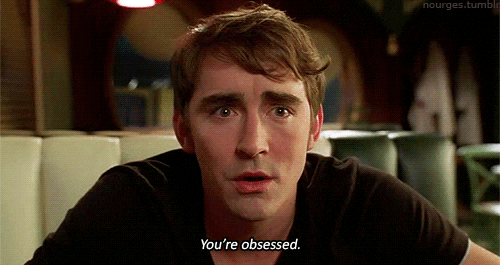# [SOLVED]Find real solutions to a system

#### anemone

##### MHB POTW Director
Staff member
Suppose that $x$ and $y$ are positive real numbers. Find all real solutions of the equation $\dfrac{2xy}{x+y}+\sqrt{\dfrac{x^2+y^2}{2}}=\sqrt{xy}+\dfrac{x+y}{2}$.

•#### topsquark

##### Well-known member
MHB Math Helper

As usual with me I can't find an efficient (or in this case even elegant) way to do this. But I had an idea...

$$\displaystyle \dfrac{2xy}{x + y} + \sqrt{ \dfrac{x^2 + y^2}{2} } = \sqrt{xy} + \dfrac{x + y}{2}$$

Let a = y/x.
Two points:
1) Watch out for x = 0 later.
2) I tacitly multiplied the first fraction by a/a. So watch out of a = 0, as well.

Then, after a bit
$$\displaystyle \dfrac{2a}{a + 1} + \dfrac{1}{ \sqrt{2} } ~ \sqrt{a^2 + 1} = \sqrt{a} + \dfrac{1}{2} (a + 1)$$

Isolate the $$\displaystyle \sqrt{a + 1}$$ and square. After some more simplifying and clearing the fractions
$$\displaystyle 2 (a^2 + 1)(a + 1)^2 = 16 a^2 + 4 a(a + 1)^2 + (a + 1)^4 - 8 a (a + 1)^2 + ( -16 a(a + 1) + 4 a (a + 1)^3 ) \sqrt{a}$$

Expanding and simplifying
$$\displaystyle 2a^4 + 4a^3 + 4a^2 + 4a + 2 = (a^4 + 14a^2 + 1) + (4a^3 - 4a^2 - 4a + 4) \sqrt{a}$$

$$\displaystyle a^4+ 4a^3 - 10a^2 + 4a + 1 = (4a^3 - 4a^2 - 4a + 4) \sqrt{a}$$

So far my approach for finding a is pretty standard. Isolate one square root, simplify, then the next step would be to isolate the other square root and simplify. But there is an issue with this procedure: If a = 1 (as it will be when we solve it) then we have both sides of this equal to 0! I have chosen to change the variable to $$\displaystyle b^2 = a$$. This means we don't have to square out that LHS, which is ugly enough. But again, we have to be careful about values of b now. (Don't worry, it'll all come together.)

So with the substitution and (yet more) simplifying:
$$\displaystyle b^8 - 4 b^7 + 4 b^6 + 4 b^5 - 10 b^4 + 4 b^3 + 4 b^2 - 4 b + 1 = 0$$

The rational root theorem says that $$\displaystyle b = \pm 1$$ are the only rational solutions. Instead of some fancy theorems to prove these are the only real solutions, I simply graphed it. It was faster.

So, $$\displaystyle b = \pm 1$$ are the only real solutions. Time to work backwards. $$\displaystyle a = b^2 = 1$$. Thus $$\displaystyle \dfrac{y}{x} = 1$$ and we get that the solutions to the original equation are all y = x. What about the x = 0 thing? y = x = 0 violates the original equation.

So the final solution is all real $$\displaystyle y = x, ~ x \neq 0$$.

-Dan

Last edited:
•MarkFL and anemone

#### anemone

##### MHB POTW Director
Staff member
Well done, topsquark ! You know, I sense your obsession lately with my POTWs and challenge problems, hehehe...I hope so far you found nothing but fun in tackling all those problems!

•topsquark and MarkFL

#### topsquark

##### Well-known member
MHB Math Helper
Well done, topsquark ! You know, I sense your obsession lately with my POTWs and challenge problems, hehehe...I hope so far you found nothing but fun in tackling all those problems!

It's not so much that I've been obsessed, it's more that I have actually been able to solve some recently. I usually try to work them out.

-Dan

•anemone and MarkFL

#### MarkFL

Staff member

It's not so much that I've been obsessed, it's more that I have actually been able to solve some recently. I usually try to work them out.

-Dan•anemone and topsquark

#### topsquark

##### Well-known member
MHB Math Helper
(Whispers) Why are we having a conversation using spoilers?

-Dan

•anemone and MarkFL

#### anemone

##### MHB POTW Director
Staff member
Because it is fun!••MarkFL and topsquark